# RD Sharma Solutions for Class 8 Maths Chapter 1 - Rational Numbers Exercise 1.3

In Exercise 1.3, we shall discuss problems based on the subtraction of rational numbers and their properties. This set of solutions are prepared by our expert tutor team to help students understand the fundamentals easily. RD Sharma Solutions for Class 8 Maths Exercise 1.3 of Chapter 1, Rational Numbers are provided here. Students can download from the links given below.

## Download PDF of RD Sharma Solutions for Class 8 Maths Exercise 1.3 Chapter 1 Rational Numbers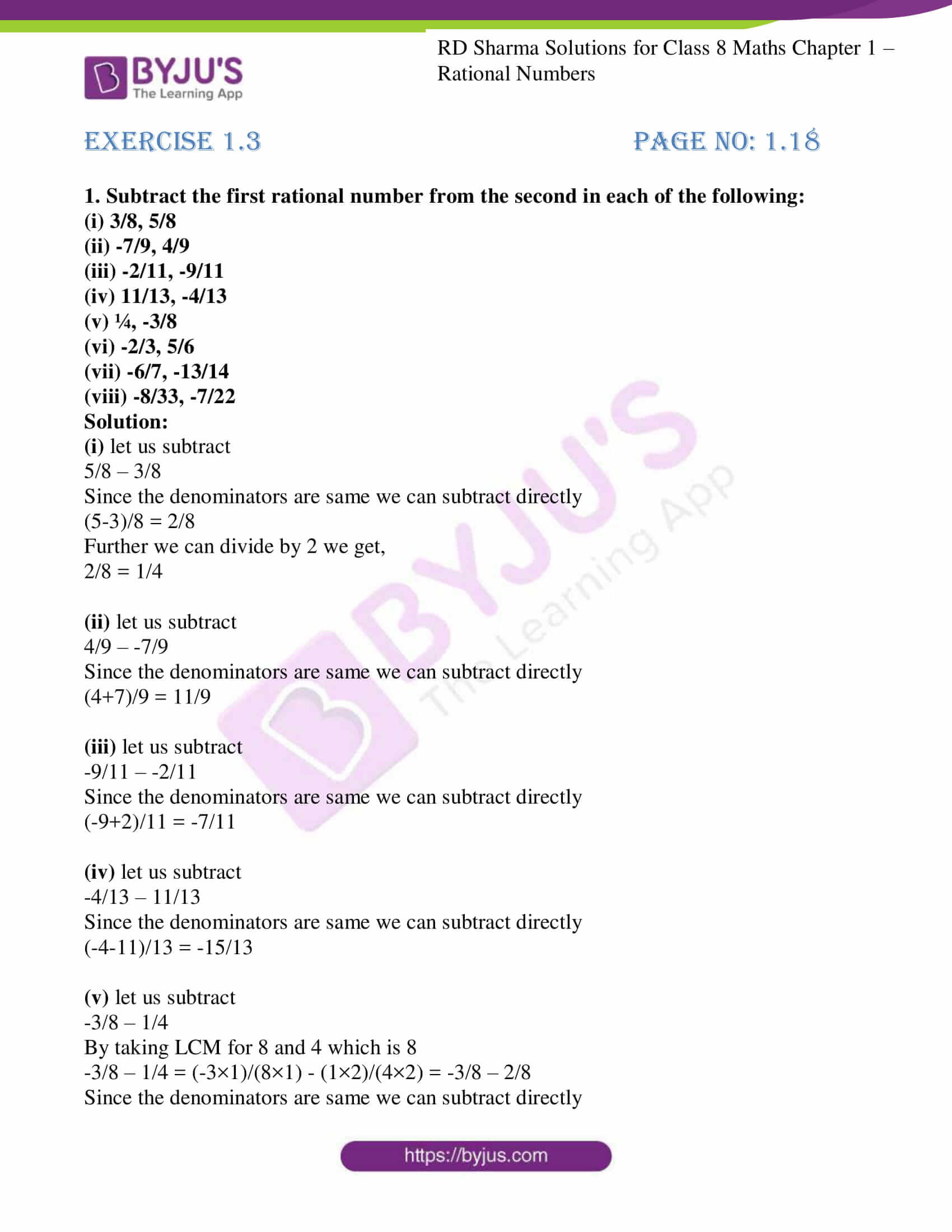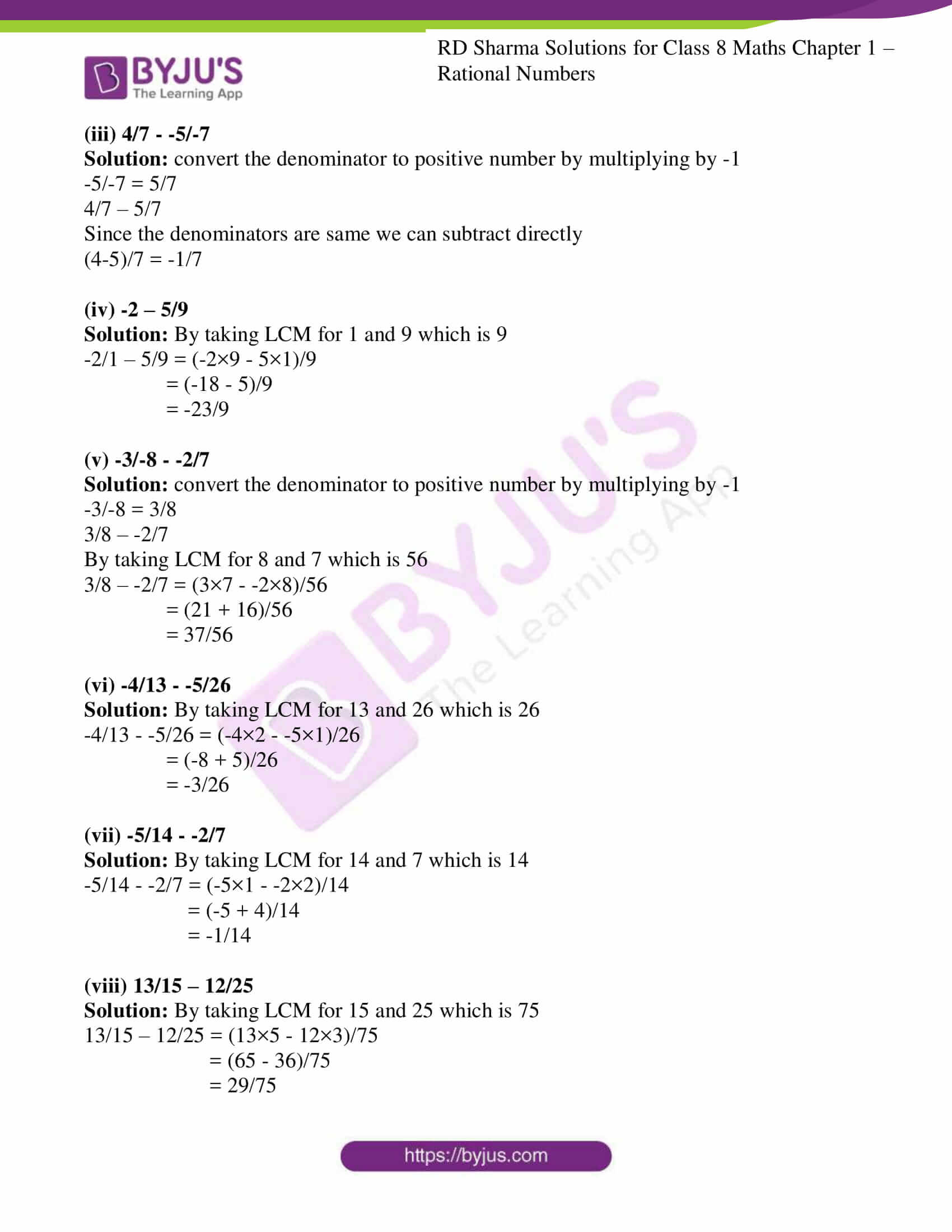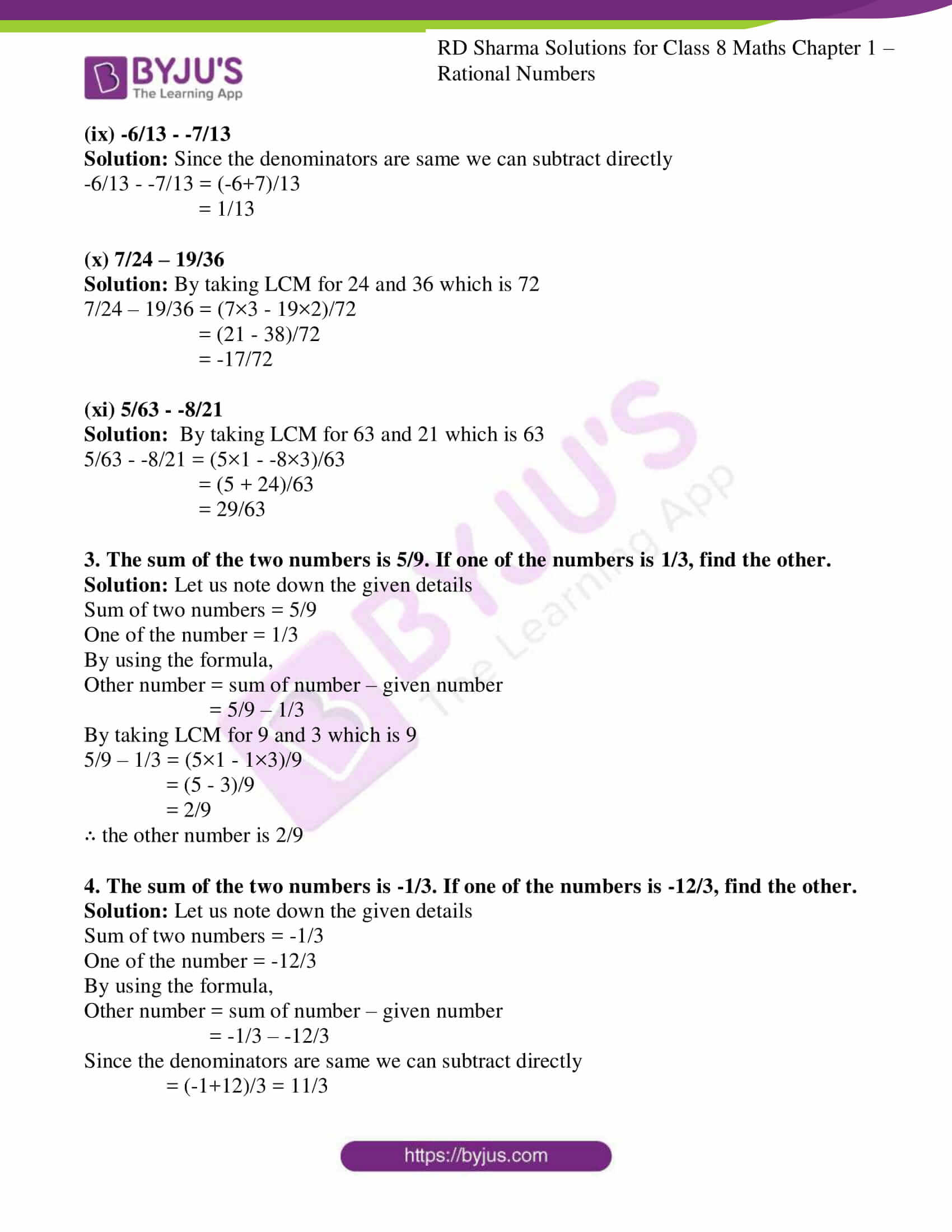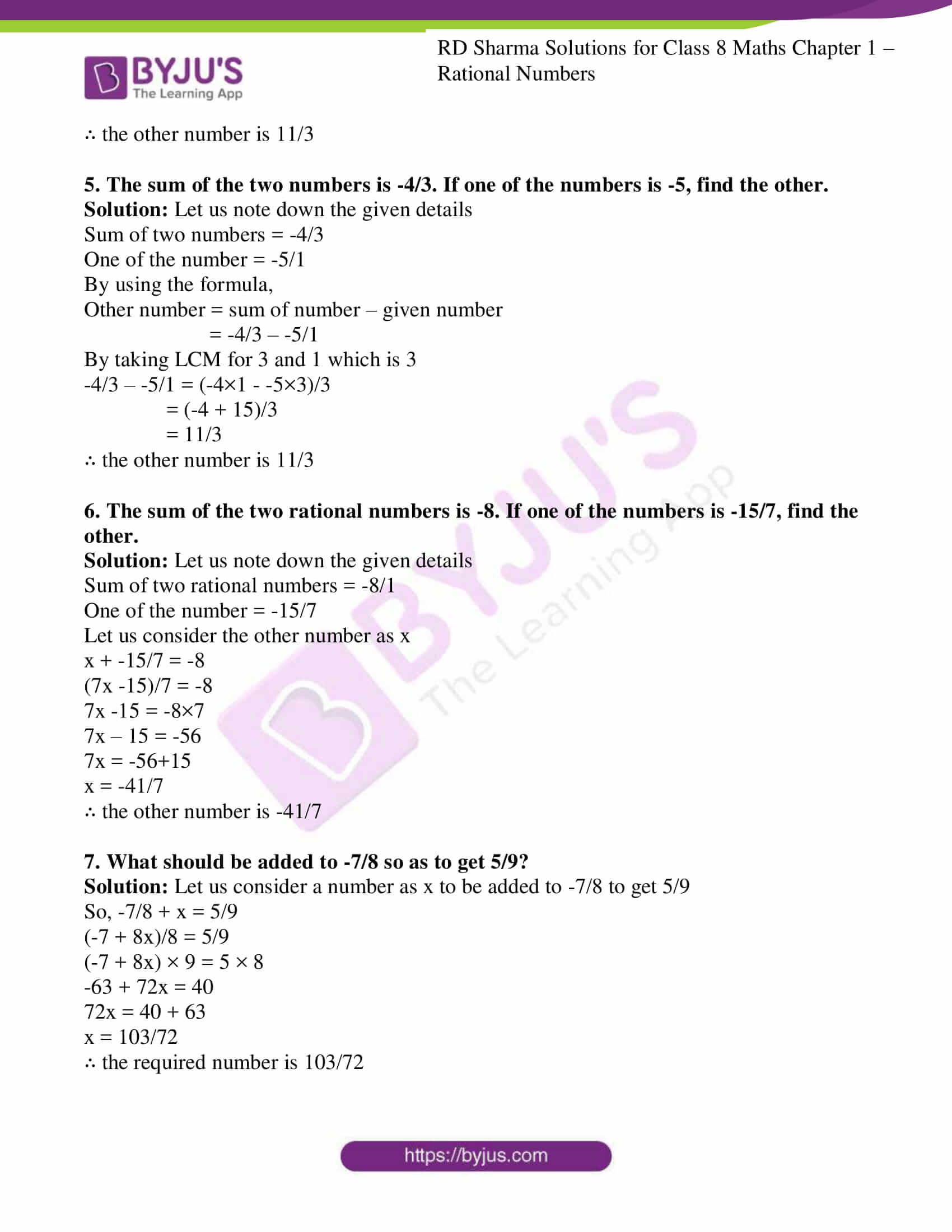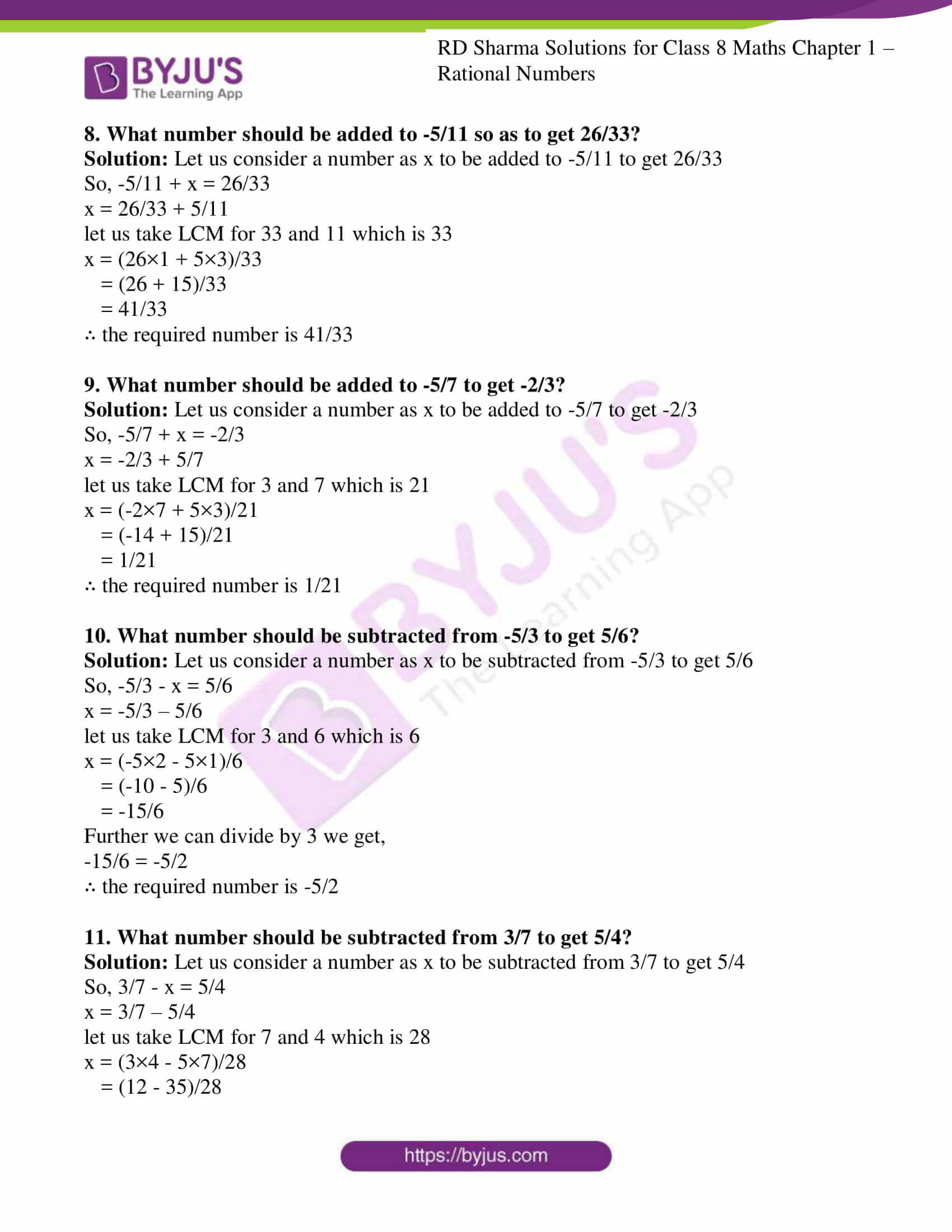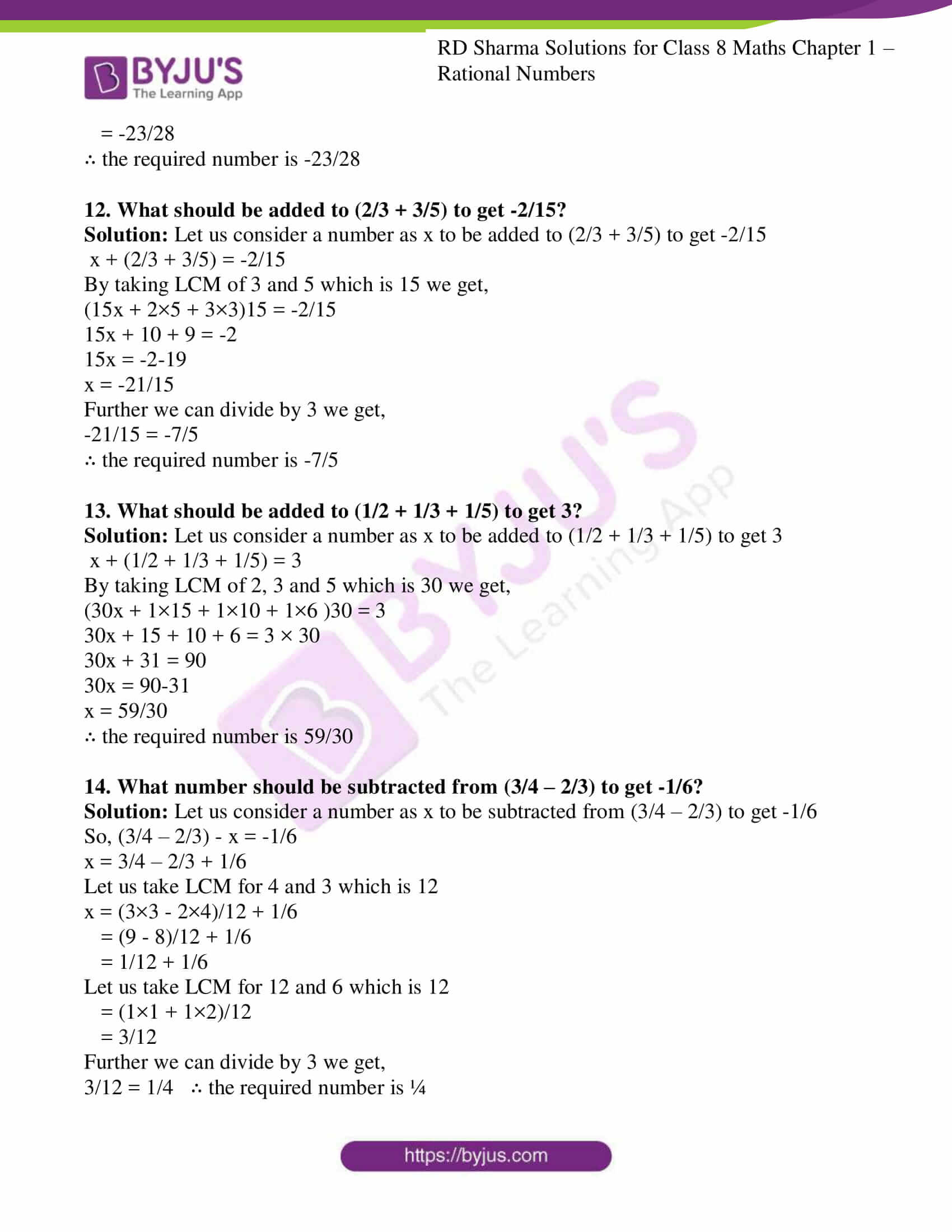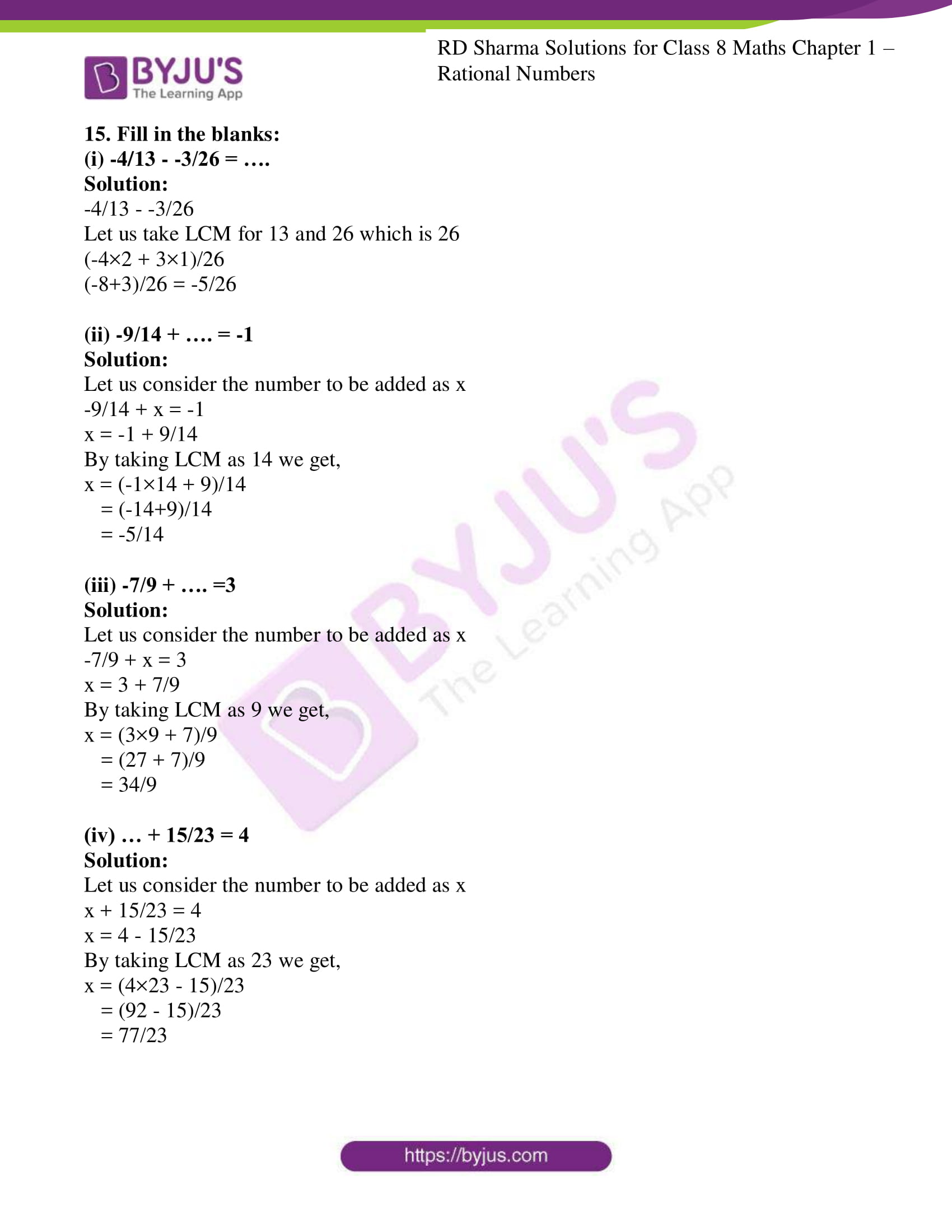### Access Answers to RD Sharma Solutions for Class 8 Maths Exercise 1.3 Chapter 1 Rational Numbers

1. Subtract the first rational number from the second in each of the following:

(i) 3/8, 5/8

(ii) -7/9, 4/9

(iii) -2/11, -9/11

(iv) 11/13, -4/13

(v) ¼, -3/8

(vi) -2/3, 5/6

(vii) -6/7, -13/14

(viii) -8/33, -7/22

Solution:

(i) let us subtract

5/8 – 3/8

Since the denominators are same we can subtract directly

(5-3)/8 = 2/8

Further we can divide by 2 we get,

2/8 = 1/4

(ii) let us subtract

4/9 – -7/9

Since the denominators are same we can subtract directly

(4+7)/9 = 11/9

(iii) let us subtract

-9/11 – -2/11

Since the denominators are same we can subtract directly

(-9+2)/11 = -7/11

(iv) let us subtract

-4/13 – 11/13

Since the denominators are same we can subtract directly

(-4-11)/13 = -15/13

(v) let us subtract

-3/8 – 1/4

By taking LCM for 8 and 4 which is 8

-3/8 – 1/4 = (-3×1)/(8×1) – (1×2)/(4×2) = -3/8 – 2/8

Since the denominators are same we can subtract directly

(-3-2)/8 = -5/8

(vi) let us subtract

5/6 – -2/3

By taking LCM for 6 and 3 which is 6

5/6 – -2/3 = (5×1)/(6×1) – (-2×2)/(3×2) = 5/6 – -4/6

Since the denominators are same we can subtract directly

(5+4)/6 = 9/6

Further we can divide by 3 we get,

9/6 = 3/2

(vii) let us subtract

-13/14 – -6/7

By taking LCM for 14 and 7 which is 14

-13/14 – -6/7 = (-13×1)/(14×1) – (-6×2)/(7×2) = -13/14 – -12/14

Since the denominators are same we can subtract directly

(-13+12)/14 = -1/14

(viii) let us subtract

-7/22 – -8/33

By taking LCM for 22 and 33 which is 66

-7/22 – -8/33 = (-7×3)/(22×3) – (-8×2)/(33×2) = -21/66 – -16/66

Since the denominators are same we can subtract directly

(-21+16)/66 = -5/66

2. Evaluate each of the following:

(i) 2/3 – 3/5

Solution: By taking LCM for 3 and 5 which is 15

2/3 – 3/5 = (2×5 – 3×3)/15

= 1/15

(ii) -4/7 – 2/-3

Solution: convert the denominator to positive number by multiplying by -1

2/-3 = -2/3

-4/7 – -2/3

By taking LCM for 7 and 3 which is 21

-4/7 – -2/3 = (-4×3 – -2×7)/21

= (-12+14)/21

= 2/21

(iii) 4/7 – -5/-7

Solution: convert the denominator to positive number by multiplying by -1

-5/-7 = 5/7

4/7 – 5/7

Since the denominators are same we can subtract directly

(4-5)/7 = -1/7

(iv) -2 – 5/9

Solution: By taking LCM for 1 and 9 which is 9

-2/1 – 5/9 = (-2×9 – 5×1)/9

= (-18 – 5)/9

= -23/9

(v) -3/-8 – -2/7

Solution: convert the denominator to positive number by multiplying by -1

-3/-8 = 3/8

3/8 – -2/7

By taking LCM for 8 and 7 which is 56

3/8 – -2/7 = (3×7 – -2×8)/56

= (21 + 16)/56

= 37/56

(vi) -4/13 – -5/26

Solution: By taking LCM for 13 and 26 which is 26

-4/13 – -5/26 = (-4×2 – -5×1)/26

= (-8 + 5)/26

= -3/26

(vii) -5/14 – -2/7

Solution: By taking LCM for 14 and 7 which is 14

-5/14 – -2/7 = (-5×1 – -2×2)/14

= (-5 + 4)/14

= -1/14

(viii) 13/15 – 12/25

Solution: By taking LCM for 15 and 25 which is 75

13/15 – 12/25 = (13×5 – 12×3)/75

= (65 – 36)/75

= 29/75

(ix) -6/13 – -7/13

Solution: Since the denominators are same we can subtract directly

-6/13 – -7/13 = (-6+7)/13

= 1/13

(x) 7/24 – 19/36

Solution: By taking LCM for 24 and 36 which is 72

7/24 – 19/36 = (7×3 – 19×2)/72

= (21 – 38)/72

= -17/72

(xi) 5/63 – -8/21

Solution: By taking LCM for 63 and 21 which is 63

5/63 – -8/21 = (5×1 – -8×3)/63

= (5 + 24)/63

= 29/63

3. The sum of the two numbers is 5/9. If one of the numbers is 1/3, find the other.

Solution: Let us note down the given details

Sum of two numbers = 5/9

One of the number = 1/3

By using the formula,

Other number = sum of number – given number

= 5/9 – 1/3

By taking LCM for 9 and 3 which is 9

5/9 – 1/3 = (5×1 – 1×3)/9

= (5 – 3)/9

= 2/9

∴ the other number is 2/9

4. The sum of the two numbers is -1/3. If one of the numbers is -12/3, find the other.

Solution: Let us note down the given details

Sum of two numbers = -1/3

One of the number = -12/3

By using the formula,

Other number = sum of number – given number

= -1/3 – -12/3

Since the denominators are same we can subtract directly

= (-1+12)/3 = 11/3

∴ the other number is 11/3

5. The sum of the two numbers is -4/3. If one of the numbers is -5, find the other.

Solution: Let us note down the given details

Sum of two numbers = -4/3

One of the number = -5/1

By using the formula,

Other number = sum of number – given number

= -4/3 – -5/1

By taking LCM for 3 and 1 which is 3

-4/3 – -5/1 = (-4×1 – -5×3)/3

= (-4 + 15)/3

= 11/3

∴ the other number is 11/3

6. The sum of the two rational numbers is -8. If one of the numbers is -15/7, find the other.

Solution: Let us note down the given details

Sum of two rational numbers = -8/1

One of the number = -15/7

Let us consider the other number as x

x + -15/7 = -8

(7x -15)/7 = -8

7x -15 = -8×7

7x – 15 = -56

7x = -56+15

x = -41/7

∴ the other number is -41/7

7. What should be added to -7/8 so as to get 5/9?

Solution: Let us consider a number as x to be added to -7/8 to get 5/9

So, -7/8 + x = 5/9

(-7 + 8x)/8 = 5/9

(-7 + 8x) × 9 = 5 × 8

-63 + 72x = 40

72x = 40 + 63

x = 103/72

∴ the required number is 103/72

8. What number should be added to -5/11 so as to get 26/33?

Solution: Let us consider a number as x to be added to -5/11 to get 26/33

So, -5/11 + x = 26/33

x = 26/33 + 5/11

let us take LCM for 33 and 11 which is 33

x = (26×1 + 5×3)/33

= (26 + 15)/33

= 41/33

∴ the required number is 41/33

9. What number should be added to -5/7 to get -2/3?

Solution: Let us consider a number as x to be added to -5/7 to get -2/3

So, -5/7 + x = -2/3

x = -2/3 + 5/7

let us take LCM for 3 and 7 which is 21

x = (-2×7 + 5×3)/21

= (-14 + 15)/21

= 1/21

∴ the required number is 1/21

10. What number should be subtracted from -5/3 to get 5/6?

Solution: Let us consider a number as x to be subtracted from -5/3 to get 5/6

So, -5/3 – x = 5/6

x = -5/3 – 5/6

let us take LCM for 3 and 6 which is 6

x = (-5×2 – 5×1)/6

= (-10 – 5)/6

= -15/6

Further we can divide by 3 we get,

-15/6 = -5/2

∴ the required number is -5/2

11. What number should be subtracted from 3/7 to get 5/4?

Solution: Let us consider a number as x to be subtracted from 3/7 to get 5/4

So, 3/7 – x = 5/4

x = 3/7 – 5/4

let us take LCM for 7 and 4 which is 28

x = (3×4 – 5×7)/28

= (12 – 35)/28

= -23/28

∴ the required number is -23/28

12. What should be added to (2/3 + 3/5) to get -2/15?

Solution: Let us consider a number as x to be added to (2/3 + 3/5) to get -2/15

x + (2/3 + 3/5) = -2/15

By taking LCM of 3 and 5 which is 15 we get,

(15x + 2×5 + 3×3)15 = -2/15

15x + 10 + 9 = -2

15x = -2-19

x = -21/15

Further we can divide by 3 we get,

-21/15 = -7/5

∴ the required number is -7/5

13. What should be added to (1/2 + 1/3 + 1/5) to get 3?

Solution: Let us consider a number as x to be added to (1/2 + 1/3 + 1/5) to get 3

x + (1/2 + 1/3 + 1/5) = 3

By taking LCM of 2, 3 and 5 which is 30 we get,

(30x + 1×15 + 1×10 + 1×6 )30 = 3

30x + 15 + 10 + 6 = 3 × 30

30x + 31 = 90

30x = 90-31

x = 59/30

∴ the required number is 59/30

14. What number should be subtracted from (3/4 – 2/3) to get -1/6?

Solution: Let us consider a number as x to be subtracted from (3/4 – 2/3) to get -1/6

So, (3/4 – 2/3) – x = -1/6

x = 3/4 – 2/3 + 1/6

Let us take LCM for 4 and 3 which is 12

x = (3×3 – 2×4)/12 + 1/6

= (9 – 8)/12 + 1/6

= 1/12 + 1/6

Let us take LCM for 12 and 6 which is 12

= (1×1 + 1×2)/12

= 3/12

Further we can divide by 3 we get,

3/12 = 1/4 ∴ the required number is ¼

15. Fill in the blanks:

(i) -4/13 – -3/26 = ….

Solution:

-4/13 – -3/26

Let us take LCM for 13 and 26 which is 26

(-4×2 + 3×1)/26

(-8+3)/26 = -5/26

(ii) -9/14 + …. = -1

Solution:

Let us consider the number to be added as x

-9/14 + x = -1

x = -1 + 9/14

By taking LCM as 14 we get,

x = (-1×14 + 9)/14

= (-14+9)/14

= -5/14

(iii) -7/9 + …. =3

Solution:

Let us consider the number to be added as x

-7/9 + x = 3

x = 3 + 7/9

By taking LCM as 9 we get,

x = (3×9 + 7)/9

= (27 + 7)/9

= 34/9

(iv) … + 15/23 = 4

Solution:

Let us consider the number to be added as x

x + 15/23 = 4

x = 4 – 15/23

By taking LCM as 23 we get,

x = (4×23 – 15)/23

= (92 – 15)/23

= 77/23

## RD Sharma Solutions for Class 8 Maths Exercise 1.3 Chapter 1 Rational Numbers

Class 8 Maths Chapter 1 Rational Numbers Exercise 1.3 is based on the subtraction of rational numbers. To facilitate easy learning and understanding of concepts download free RD Sharma Solutions Chapter 1 in PDF format, which provides answers to all the questions. Practising as many times as possible helps students in building time management skills and also boosts the confidence level to achieve high marks.